# Back to School Night

Tuesday, September 10
6:30 - 8:40 pm

What goes on in your daughter’s Latin class? Who is that interesting world studies teacher your son talks about?

Find out the answers to these questions and more during our annual Back to School Night.

Arrive by 6:15 pm to pick up your personalized schedule.

Back to School Night is a great opportunity for you to follow your child's schedule, meet your child's teachers, and learn about teacher expectations, and classroom activities. (Please note, this is not the time to discuss your student's progress with the teachers.)

For more information, click HERE.

 table div table+table+table div table{width:100%;padding:0}table div table+table+table div table img{width:96.23%;padding:0;float:none}table div table+table+table div table td{width:100%;padding:0 1.88% 18px}/* styles */# Volunteers Needed for Back to School Night

Volunteers are needed to pick up coffee and cookies, to set up the refreshment serving tables, and to clean up afterward. Coffee and cookie purchases can be reimbursed or considered charitable donations to Friends of Northside. Sign up HERE.

 table div table+table+table+table+table div table{width:100%;padding:0}table div table+table+table+table+table div table img{width:96.23%;padding:0;float:none}table div table+table+table+table+table div table td{width:100%;padding:0 1.88% 18px}/* styles */# Introduction: Chris Mourtokokis (New NCP School Counselor)

Greetings Mustangs!

My name is Chris Mourtokokis, but many students simply call me Mourty. I am the new school counselor here at Northside College Prep.

I was a counselor for three years at Cary-Grove High School and three years at St. Patrick High School. I graduated from DePaul University with a major in psychology. I then went on to earn my master's in School Counseling from National Louis University, where I also became a Licensed Professional Counselor and a National Board Certified Counselor. In addition, I just completed my master's in Educational Leadership from Northeastern Illinois University.

I am so excited to get to know everyone and be a part of the NCP community! I am looking forward to working with all of you.

Thank you, and GO MUSTANGS!

 table div table+table+table+table+table+table+table div table{width:100%;padding:0}table div table+table+table+table+table+table+table div table img{width:96.23%;padding:0;float:none}table div table+table+table+table+table+table+table div table td{width:100%;padding:0 1.88% 18px}/* styles */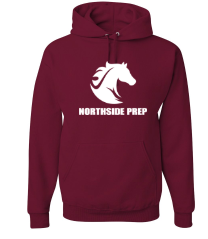# Northside Mustangs Spirit Wear Store

The Athletic Boosters have coordinated with All Seasons Screen Printing & Embroidery again this year to make NCP Spirit wear available to everyone online. The new mustang logo has now been incorporated into the offerings from last year. Shop for the latest gear HERE.

The store will be open until 11:59 pm on Sunday, September 29. Get your spirit wear today!

 table div table+table+table+table+table+table+table+table+table+table div table{width:100%;padding:0}table div table+table+table+table+table+table+table+table+table+table div table img{width:96.23%;padding:0;float:none}table div table+table+table+table+table+table+table+table+table+table div table td{width:100%;padding:0 1.88% 18px}/* styles */# Parking Lot Safety

Our NCP school parking lot is a bustling place before and after school. Families dropping off and picking up students are asked to be mindful of both safety and courtesy. Please assist us by ensuring that traffic lanes stay clear during drop-off and pick-up times and by following the parking lot procedures.

# Parking Lot Procedures

ALL vehicles MUST exit onto Kedzie. You may enter the parking lot from Kedzie or Bryn Mawr, but regardless of where you enter, all cars must exit onto Kedzie. For the safety of our students and the rest of the Northside community, please do not exit onto Bryn Mawr.

The first few days will be busy at drop-off and pick-up. Please, for the safety of all involved, plan to give yourself enough time for drop-off and pick-up and please be patient while in the parking lot. Pulling over to the curb on the right as your student enters or exits your vehicle keeps students safe and traffic flow efficient. Our safety team will be actively working to ensure that students arrive and depart safely each day and that vehicles in school lots remain damage free.

 table div table+table+table+table+table+table+table+table+table+table+table+table div table{width:100%;padding:0}table div table+table+table+table+table+table+table+table+table+table+table+table div table img{width:96.23%;padding:0;float:none}table div table+table+table+table+table+table+table+table+table+table+table+table div table td{width:100%;padding:0 1.88% 18px}/* styles */# CPS Volunteer Requirements

According to CPS policy, anybody who is going to be in contact with students must be approved as a CPS volunteer. There are two levels of volunteers, Level 1 and Level 2.

## Level I Volunteers

(Please allow 8-12 weeks for the entire process)
A fingerprint criminal background check is required for all Level I Volunteers.

A Level I volunteer is:

1. A parent providing volunteer service in their child’s school or classroom for 10 or more hours/week;
2. An individual providing volunteer service for 5 or more hours/week at a school where s/he does not have a child enrolled;
3. Any individual chaperoning an overnight school-sponsored trip, regardless of the hours/week that the volunteer serves (See also the Student Travel Policy).
 1 A parent providing volunteer service in their child’s school or classroom for 10 or more hours/week;
 2 An individual providing volunteer service for 5 or more hours/week at a school where s/he does not have a child enrolled;
 3 Any individual chaperoning an overnight school-sponsored trip, regardless of the hours/week that the volunteer serves (See also the Student Travel Policy).

## Level II Volunteers

(This process can be completed in a day & is required even of volunteers for Friends of Northside events)
A fingerprint criminal background check is not required for Level II Volunteers.

A Level II Volunteer is:

1. A parent providing supervised volunteer service in their child’s school or classroom for less than 10 hours/week;
2. Any individual providing volunteer service for less than 5 hours/week at a school where s/he does not have a child enrolled;
3. An individual providing incidental volunteer service with no ongoing individualized interaction with a student(s) including those who speak at a class/assembly, judge academic competitions, give musical performance, participate in the “Principal for a Day” program, job shadowing event or other one-time event provided where there is direct supervision of the activity/event by regular school employees;
4. A parent accompanying his/her child’s class on a one-day field trip or another type of extracurricular activity that does not involve an overnight stay;
5. An individual providing volunteer service on projects/activities involving no or nominal contact with children (home-based volunteer activities, volunteers serving at Central or Network office).
 1 A parent providing supervised volunteer service in their child’s school or classroom for less than 10 hours/week;
 2 Any individual providing volunteer service for less than 5 hours/week at a school where s/he does not have a child enrolled;
 3 An individual providing incidental volunteer service with no ongoing individualized interaction with a student(s) including those who speak at a class/assembly, judge academic competitions, give musical performance, participate in the “Principal for a Day” program, job shadowing event or other one-time event provided where there is direct supervision of the activity/event by regular school employees;
 4 A parent accompanying his/her child’s class on a one-day field trip or another type of extracurricular activity that does not involve an overnight stay;
 5 An individual providing volunteer service on projects/activities involving no or nominal contact with children (home-based volunteer activities, volunteers serving at Central or Network office).

To become a volunteer, please visit CPS' Volunteer website and follow the instructions. There is also a link on Northside's website on the Parents page Northside Volunteers.

Assistant Principal, Kaitlin O'Hare is Northside's Volunteer Coordinator. After applying to become a volunteer, you must visit the school and show Ms. O'Hare your ID, so she can approve you to move forward in the volunteer process. For Level II volunteers, that is the only step you must take. For Level 1, you will receive further instructions from CPS.

 table div table+table+table+table+table+table+table+table+table+table+table+table+table+table div table{width:100%;padding:0}table div table+table+table+table+table+table+table+table+table+table+table+table+table+table div table img{width:96.23%;padding:0;float:none}table div table+table+table+table+table+table+table+table+table+table+table+table+table+table div table td{width:100%;padding:0 1.88% 18px}/* styles */# This Week in Sports

Fall sports have begun. Come out and support our student athletes!

## Women's Tennis

▪ Monday, September 9, 5:00 pm (V) vs. Lincoln Park at Oz Park
▪ Tuesday, September 10, 5:00 pm (V) vs. Whitney Young at NCP
▪ Thursday, September 12, 5:00 pm (V) vs. Walter Payton College Prep at Oz Park
 ▪ Monday, September 9, 5:00 pm (V) vs. Lincoln Park at Oz Park
 ▪ Tuesday, September 10, 5:00 pm (V) vs. Whitney Young at NCP
 ▪ Thursday, September 12, 5:00 pm (V) vs. Walter Payton College Prep at Oz Park

## Men's Soccer

▪ Monday, September 9, 4:30 pm (JV) and 6:30 pm (V) vs. Notre Dame College Prep at Notre Dame College Prep
▪ Wednesday, September 11, 4:30 pm (S) vs. Whitney Young at NCP
▪ Wednesday, September 11, 4:30 pm (V) and 6:00 pm (JV) vs. Sullivan at Winnemac Stadium
▪ Thursday, September 12, 4:30 pm (V) and 6:00 pm (JV) vs. Lane Tech at NCP
 ▪ Monday, September 9, 4:30 pm (JV) and 6:30 pm (V) vs. Notre Dame College Prep at Notre Dame College Prep
 ▪ Wednesday, September 11, 4:30 pm (S) vs. Whitney Young at NCP
 ▪ Wednesday, September 11, 4:30 pm (V) and 6:00 pm (JV) vs. Sullivan at Winnemac Stadium
 ▪ Thursday, September 12, 4:30 pm (V) and 6:00 pm (JV) vs. Lane Tech at NCP

## Women's Volleyball

▪ Tuesday, September 10, 5:00 pm (F) and 6:00 pm (F-B) vs. Chicago Amundsen at Chicago Amundsen High School
▪ Tuesday, September 10, 5:00 pm (V) and 6:00 pm (JV) vs. Whitney Young at NCP
▪ Thursday, September 12, 5:00 pm (V) and 6:00 pm (JV) vs. Lincoln Park at Lincoln Park High School
▪ Thursday, September 12, TBD (V) vs. Niles West at Niles West High School
▪ Saturday, September 14, TBD (V) and (JV) vs. Niles West at Niles West High School
 ▪ Tuesday, September 10, 5:00 pm (F) and 6:00 pm (F-B) vs. Chicago Amundsen at Chicago Amundsen High School
 ▪ Tuesday, September 10, 5:00 pm (V) and 6:00 pm (JV) vs. Whitney Young at NCP
 ▪ Thursday, September 12, 5:00 pm (V) and 6:00 pm (JV) vs. Lincoln Park at Lincoln Park High School
 ▪ Thursday, September 12, TBD (V) vs. Niles West at Niles West High School
 ▪ Saturday, September 14, TBD (V) and (JV) vs. Niles West at Niles West High School

## Women's Softball

▪ Monday, September 9, 4:30 pm (V) vs. Chicago Academy at NCP
▪ Wednesday, September 11, 4:30 pm (V) vs. Lake View at Lake View High School
 ▪ Monday, September 9, 4:30 pm (V) vs. Chicago Academy at NCP
 ▪ Wednesday, September 11, 4:30 pm (V) vs. Lake View at Lake View High School

## Women's Swimming

▪ Monday, September 9, 5:00 pm (V) vs. Lincoln Park at NCP
 ▪ Monday, September 9, 5:00 pm (V) vs. Lincoln Park at NCP

## Women's Golf

▪ Monday, September 9, 4:00 pm (V) vs. Chicago (Kenwood), Chicago (Lindblom) at Columbus Golf Course
▪ Wednesday, September 11, 4:00 pm (V) vs. Chicago (Taft), Lake View at Billy Caldwell Golf Course
▪ Saturday, September 14, 11:00 am (V) vs. TBA at Jackson Park Golf Course
 ▪ Monday, September 9, 4:00 pm (V) vs. Chicago (Kenwood), Chicago (Lindblom) at Columbus Golf Course
 ▪ Wednesday, September 11, 4:00 pm (V) vs. Chicago (Taft), Lake View at Billy Caldwell Golf Course
 ▪ Saturday, September 14, 11:00 am (V) vs. TBA at Jackson Park Golf Course

## Men's Golf

▪ Tuesday, September 10, 3:30 pm (V) vs. Lane Tech at Billy Caldwell Golf Course
▪ Thursday, September 12, 3:30 pm (V) vs. Chicago (Kenwood), Chicago (Taft) at Columbus Golf Course
▪ Saturday, September 14, 11:00 am (V) vs. TBA at Jackson Park Golf Course
 ▪ Tuesday, September 10, 3:30 pm (V) vs. Lane Tech at Billy Caldwell Golf Course
 ▪ Thursday, September 12, 3:30 pm (V) vs. Chicago (Kenwood), Chicago (Taft) at Columbus Golf Course
 ▪ Saturday, September 14, 11:00 am (V) vs. TBA at Jackson Park Golf Course

## Women's Cross Country

▪ Wednesday, September 11, 4:30 pm (V) vs. Steinmetz College Prep at Riis Park
▪ Saturday, September 14, 9:00 am (V) vs. First to the Finish at Detweiller Park
 ▪ Wednesday, September 11, 4:30 pm (V) vs. Steinmetz College Prep at Riis Park
 ▪ Saturday, September 14, 9:00 am (V) vs. First to the Finish at Detweiller Park

## Men's Cross Country

▪ Wednesday, September 11, 4:30 pm (V) vs. Steinmetz College Prep at Riis Park
▪ Saturday, September 14, 9:00 am (V) vs. First to the Finish at Detweiller Park
 ▪ Wednesday, September 11, 4:30 pm (V) vs. Steinmetz College Prep at Riis Park
 ▪ Saturday, September 14, 9:00 am (V) vs. First to the Finish at Detweiller Park
 /* styles */ Visit the NCP Athletics website for additional information about Northside teams.
 table div table+table+table+table+table+table+table+table+table+table+table+table+table+table+table+table+table div table{width:100%;padding:0}table div table+table+table+table+table+table+table+table+table+table+table+table+table+table+table+table+table div table img{width:96.23%;padding:0;float:none}table div table+table+table+table+table+table+table+table+table+table+table+table+table+table+table+table+table div table td{width:100%;padding:0 1.88% 18px}/* styles */# Get Involved!

We encourage parents to be an active part of the Northside community. All are welcome to attend Friends of Northside and Local School Council meetings. Monthly meeting dates are posted on the home page of the school website under Upcoming Events.

 table div table+table+table+table+table+table+table+table+table+table+table+table+table+table+table+table+table+table+table div table{width:100%;padding:0}table div table+table+table+table+table+table+table+table+table+table+table+table+table+table+table+table+table+table+table div table img{width:96.23%;padding:0;float:none}table div table+table+table+table+table+table+table+table+table+table+table+table+table+table+table+table+table+table+table div table td{width:100%;padding:0 1.88% 18px}/* styles */# Local School Council (LSC)

The Local School Council (LSC) - the voice in the continuing evolution of Northside - is an elected body that plays an important role in the decision-making that affects our school. The LSC is responsible for approving the Continuous Improvement Work Plan (CIWP), principal selection and evaluation, and allocating discretionary funds to school programs. The next LSC meeting is Tuesday, September 17 at 6:30 pm in the Faculty Lounge.

You can learn more about the Local School Council HERE.

 table div table+table+table+table+table+table+table+table+table+table+table+table+table+table+table+table+table+table+table+table+table div table{width:100%;padding:0}table div table+table+table+table+table+table+table+table+table+table+table+table+table+table+table+table+table+table+table+table+table div table img{width:96.23%;padding:0;float:none}table div table+table+table+table+table+table+table+table+table+table+table+table+table+table+table+table+table+table+table+table+table div table td{width:100%;padding:0 1.88% 18px}/* styles */# Friends of Northside

Friends of Northside (FON) is the “one stop shop” for NCP families to get involved. FON facilitates communication between parents and the school, organizes parent volunteers, plans and sponsors social/educational events, and raises much-needed funds to bridge the gap due to CPS budget cuts. FON supports the school’s mission to maintain and enhance the learning experience for all students of Northside College Prep High School. The next FON meeting will be Tuesday, September 24 at 7:00 pm in the Faculty Lounge.

To find out more about Friends of Northside, please visit the webpage HERE.

 table div table+table+table+table+table+table+table+table+table+table+table+table+table+table+table+table+table+table+table+table+table+table+table div table{width:100%;padding:0}table div table+table+table+table+table+table+table+table+table+table+table+table+table+table+table+table+table+table+table+table+table+table+table div table img{width:96.23%;padding:0;float:none}table div table+table+table+table+table+table+table+table+table+table+table+table+table+table+table+table+table+table+table+table+table+table+table div table td{width:100%;padding:0 1.88% 18px}/* styles */# Fall Parents Night Out

Thursday, September 26
6:00 pm
Via Veneto
6340 N. Lincoln Ave

Join us for a Parents' Night Out at Via Veneto. There will be complimentary appetizers and a cash bar. Catch up with old friends and make new ones! Please sign up HERE so we can let the restaurant know how many people to expect.

 table div table+table+table+table+table+table+table+table+table+table+table+table+table+table+table+table+table+table+table+table+table+table+table+table+table+table div table{width:100%;padding:0}table div table+table+table+table+table+table+table+table+table+table+table+table+table+table+table+table+table+table+table+table+table+table+table+table+table+table div table img{width:96.23%;padding:0;float:none}table div table+table+table+table+table+table+table+table+table+table+table+table+table+table+table+table+table+table+table+table+table+table+table+table+table+table div table td{width:100%;padding:0 1.88% 18px}/* styles */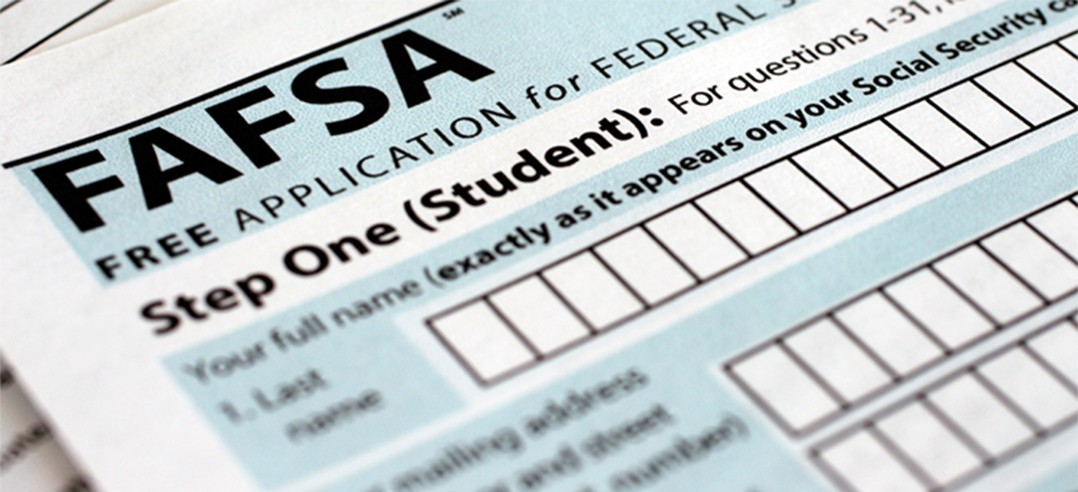# Financial Aid Completion Workshops

Tuesday, October 15
6:00 - 8:00 pm

The counseling department will be offering FAFSA Completion Workshops for English and Spanish speaking parents and students on Tuesday, October 15 from 6:00 - 8:00 pm. Additional student workshops will be held during the day for students who would like to start their FAFSA as soon as the application becomes available or if they would like one on one support.

Students can register for the sessions offered during the school day in their Enriching Student Portal.

## Presentations

ISAC Student FAFSA Workshop
Wednesday, October 2
Room 224
Two sessions: 1:25 - 2:10 pm and 2:15 - 3:00 pm

ISAC Parent and Student FAFSA Workshop (English & Spanish)
Tuesday, October 16
6:00 - 8:00 pm
Room 219

ISAC Student Financial Aid Follow-up
Wednesday, November 20
Room 224
Two sessions: 1:25 - 2:10 pm and 2:15 - 3:00 pm

 table div table+table+table+table+table+table+table+table+table+table+table+table+table+table+table+table+table+table+table+table+table+table+table+table+table+table+table+table div table{width:100%;padding:0}table div table+table+table+table+table+table+table+table+table+table+table+table+table+table+table+table+table+table+table+table+table+table+table+table+table+table+table+table div table img{width:96.23%;padding:0;float:none}table div table+table+table+table+table+table+table+table+table+table+table+table+table+table+table+table+table+table+table+table+table+table+table+table+table+table+table+table div table td{width:100%;padding:0 1.88% 18px}/* styles */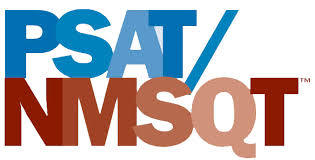# PSAT/NMST Exam

Wednesday, October 16
8:00 am

Northside College Prep sophomores and juniors will participate in the PSAT/NMSQT exam. On the day of the exam, students are expected to report to school no later than 8:00 am for a full day of school. More details about the exam will be shared with students and parents in the near future.

 table div table+table+table+table+table+table+table+table+table+table+table+table+table+table+table+table+table+table+table+table+table+table+table+table+table+table+table+table+table+table div table{width:100%;padding:0}table div table+table+table+table+table+table+table+table+table+table+table+table+table+table+table+table+table+table+table+table+table+table+table+table+table+table+table+table+table+table div table img{width:96.23%;padding:0;float:none}table div table+table+table+table+table+table+table+table+table+table+table+table+table+table+table+table+table+table+table+table+table+table+table+table+table+table+table+table+table+table div table td{width:100%;padding:0 1.88% 18px}/* styles */# Academic Approach: PSAT Prep Schedule

Academic Approach is offering a standardized test preparation course for Northside College Prep students who have targeted the October 16th, 2019 PSAT/NMSQT exam. This course is best suited for students who are interested in an efficient preparation course reviewing key skills in reading, writing, math, and science and their application on the SAT. The 15 hours of total instruction introduces reading annotation, math problem solving, and grammar and rhetorical skills, all with rigorous emphasis on SAT standards and practices.

As part of the course, each student will receive a full-length practice test. It is scheduled within the course. Students will receive their practice test results within a week of taking the exam. Please see the schedule below for a weekly breakdown of classes.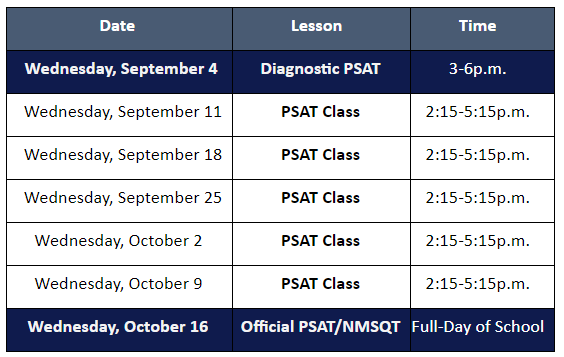table div table+table+table+table+table+table+table+table+table+table+table+table+table+table+table+table+table+table+table+table+table+table+table+table+table+table+table+table+table+table+table+table+table div table{width:100%;padding:0}table div table+table+table+table+table+table+table+table+table+table+table+table+table+table+table+table+table+table+table+table+table+table+table+table+table+table+table+table+table+table+table+table+table div table img{width:96.23%;padding:0;float:none}table div table+table+table+table+table+table+table+table+table+table+table+table+table+table+table+table+table+table+table+table+table+table+table+table+table+table+table+table+table+table+table+table+table div table td{width:100%;padding:0 1.88% 18px}/* styles */# No Experience Necessary

Make a commitment to Northside by offering your time for any of these rewarding on-going volunteer positions. Email Friends of Northside to indicate your interest or ask questions.

## Graphic Designer

Provide professional guidance and materials for various design needs throughout the year.

## Volunteer Coordinator

Manage and update our online volunteer system as needed throughout the year.

## Spring Benefit Committee

The Spring Benefit is NCP’s major spring fundraiser. Get involved, get to know NCP staff and parents, and make a profound impact on Northside College Prep.

When is the Spring Benefit?
Friday, March 13, 2020

What’s the time commitment for the team?
Meetings begin in the middle of September. The time commitment depends on your role. There is something for everyone. Please join us!

What roles are available?
* Entertainment Chair--one or two people to determine and arrange the entertainment for the Spring Benefit.
* Auction Item Solicitors--at least five people to solicit auction items.
* Printed Materials Chair--one or two people to design and produce all the printed materials.

Email debra@theswans.net for more information or to join the team!

 table div table+table+table+table+table+table+table+table+table+table+table+table+table+table+table+table+table+table+table+table+table+table+table+table+table+table+table+table+table+table+table+table+table+table+table+table+table div table{width:100%;padding:0}table div table+table+table+table+table+table+table+table+table+table+table+table+table+table+table+table+table+table+table+table+table+table+table+table+table+table+table+table+table+table+table+table+table+table+table+table+table div table img{width:96.23%;padding:0;float:none}table div table+table+table+table+table+table+table+table+table+table+table+table+table+table+table+table+table+table+table+table+table+table+table+table+table+table+table+table+table+table+table+table+table+table+table+table+table div table td{width:100%;padding:0 1.88% 18px}/* styles */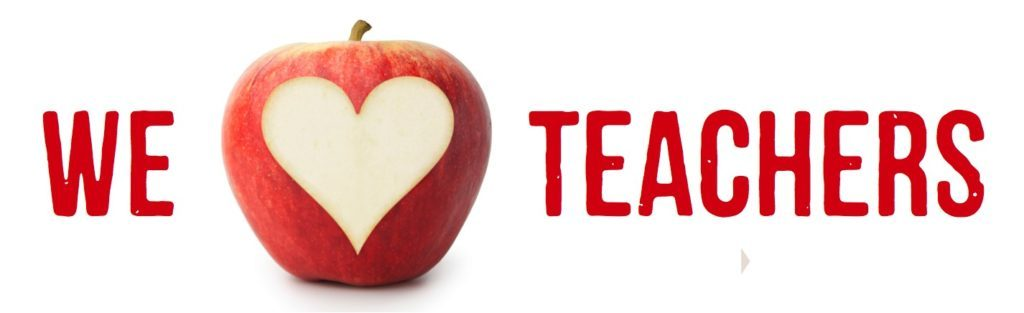# Help Needed With the Teachers' Lounge

We are looking for one additional helper to keep the Teachers' Lounge stocked with coffee, tea, etc. We're looking for someone who can come on Wednesdays. It takes about 30 minutes and the time is flexible. It's a great way to get involved in your child's school! Contact Eileen at 617.642.6267 for details.

 table div table+table+table+table+table+table+table+table+table+table+table+table+table+table+table+table+table+table+table+table+table+table+table+table+table+table+table+table+table+table+table+table+table+table+table+table+table+table+table+table div table{width:100%;padding:0}table div table+table+table+table+table+table+table+table+table+table+table+table+table+table+table+table+table+table+table+table+table+table+table+table+table+table+table+table+table+table+table+table+table+table+table+table+table+table+table+table div table img{width:96.23%;padding:0;float:none}table div table+table+table+table+table+table+table+table+table+table+table+table+table+table+table+table+table+table+table+table+table+table+table+table+table+table+table+table+table+table+table+table+table+table+table+table+table+table+table+table div table td{width:100%;padding:0 1.88% 18px}/* styles */# Do you have news or team results to share with the Northside parent community?

## Click HERE to submit information.

 table div table+table+table+table+table+table+table+table+table+table+table+table+table+table+table+table+table+table+table+table+table+table+table+table+table+table+table+table+table+table+table+table+table+table+table+table+table+table+table+table+table+table+table div table{width:100%;padding:0}table div table+table+table+table+table+table+table+table+table+table+table+table+table+table+table+table+table+table+table+table+table+table+table+table+table+table+table+table+table+table+table+table+table+table+table+table+table+table+table+table+table+table+table div table img{width:96.23%;padding:0;float:none}table div table+table+table+table+table+table+table+table+table+table+table+table+table+table+table+table+table+table+table+table+table+table+table+table+table+table+table+table+table+table+table+table+table+table+table+table+table+table+table+table+table+table+table div table td{width:100%;padding:0 1.88% 18px}/* styles */# September College Visits at Northside

Monday, September 9
Bowling Green State University, 10:15 am
University College Dublin, 10:15 am
Eastern Illinois University, 10:15 am
University of Missouri Columbia, 10:15 am
CCC, Harold Washington College, 10:38 am
Antioch College, 11:36 am
Kent State University, 11:36 am

Tuesday, September 10
Bucknell University, 9:48 am
Gettysburg College, 9:48 am
University of San Francisco, 9:48 am
California Polytechnic State Univ, San Luis Obispo, 10:38 am
Cornell College, 11:36 am

Wednesday, September 11
Johns Hopkins University, 10:40 am
Purdue University Northwest, 10:40 am
Brown University, 11:20 am
Tulane University, 11:20 am
Yale University, 1:25 pm
Bentley University, 3:15 pm

Thursday, September 12
North Park University, 11:36 am
Carnegie Mellon University, 3:15 pm

Friday, September 13
Eureka College, 11:36 am
Holy Cross College, 3:15 pm

Monday, September 16
University of California, Santa Cruz, 9:48 am
University of St. Francis, 9:48 am
Syracuse University, 9:48 am
Sarah Lawrence College, 11:36 am
Stetson University, 11:36 am
Case Western Reserve University, 12:26 pm
Emory University, 12:26 pm
University of Michigan, 3:15 pm

Tuesday, September 17
Columbia College Chicago, 9:48 am
Hillsdale College, 9:48 am
College of the Holy Cross, 9:48 am
Saint Mary's College, 9:48 am
Columbia University International Dual BA Programs, 11:36 am
Tufts University, 11:36 am
University of Aberdeen, 12:26 pm
University of Southern California, 3:15 pm

Wednesday, September 18
University of Illinois at Urbana-Champaign, 10:40 am
Chicago State University, 11:20 pm
IE University - Segovia, 11:20 am
Chicago State University, 12:40 pm
Louisiana State University, 12:40 pm
The College of Wooster, 12:40 pm
John Carroll University, 3:15 pm

Thursday, September 19
Southern Illinois University, Carbondale, 9:48 am
Washington University in St. Louis, 9:48 am
Knox College, 10:38 am
University of Notre Dame, 10:38 am
Temple University Japan, 12:26 pm
Michigan State University, 3:15 pm

Friday, September 20
Reed College, 9:48 am
Rollins College, 9:48 am
Boston University, 11:36 am
Southern Illinois University Edwardsville, 12:26 pm

Monday, September 23
Massachusetts Institute of Technology, 3:15 pm

Tuesday, September 24
St. John's University, 10:38 am
Saint Louis University, 12:26 pm

Wednesday, September 25
Suffolk University, 11:20 am
Kalamazoo College, 1:25 pm
Indiana University at Bloomington, 3:15 pm

Thursday, September 26
DePauw University, 9:48 am
Missouri State University, 12:26 pm
American University, 3:15 pm

Monday, September 30
Barnard College, 9:48 am
University of California, Berkeley, 9:48 am
Middlebury College, 9:48 am
Wesleyan University, 10:38 am
Lehigh University, 11:36 am
Temple University, 3:15 pm

The list of college visits is updated frequently. For the most current information, parents of juniors and seniors should log into their parent account for Naviance.

Students are highly encouraged to meet with the reps who take the time to visit Northside. Oftentimes these are the same regional reps who will be reading your child's application! Students may ask a teacher to be briefly excused from class to meet a college representative.

 table div table+table+table+table+table+table+table+table+table+table+table+table+table+table+table+table+table+table+table+table+table+table+table+table+table+table+table+table+table+table+table+table+table+table+table+table+table+table+table+table+table+table+table+table+table+table div table{width:100%;padding:0}table div table+table+table+table+table+table+table+table+table+table+table+table+table+table+table+table+table+table+table+table+table+table+table+table+table+table+table+table+table+table+table+table+table+table+table+table+table+table+table+table+table+table+table+table+table+table div table img{width:96.23%;padding:0;float:none}table div table+table+table+table+table+table+table+table+table+table+table+table+table+table+table+table+table+table+table+table+table+table+table+table+table+table+table+table+table+table+table+table+table+table+table+table+table+table+table+table+table+table+table+table+table+table div table td{width:100%;padding:0 1.88% 18px}/* styles */# CPS Scholarship Notifications and College Events Around the City

For more details about the upcoming events and to access the most recent updates, view the College Calendar on the Northside Prep Counseling Website HERE.

 table div table+table+table+table+table+table+table+table+table+table+table+table+table+table+table+table+table+table+table+table+table+table+table+table+table+table+table+table+table+table+table+table+table+table+table+table+table+table+table+table+table+table+table+table+table+table+table+table div table{width:100%;padding:0}table div table+table+table+table+table+table+table+table+table+table+table+table+table+table+table+table+table+table+table+table+table+table+table+table+table+table+table+table+table+table+table+table+table+table+table+table+table+table+table+table+table+table+table+table+table+table+table+table div table img{width:96.23%;padding:0;float:none}table div table+table+table+table+table+table+table+table+table+table+table+table+table+table+table+table+table+table+table+table+table+table+table+table+table+table+table+table+table+table+table+table+table+table+table+table+table+table+table+table+table+table+table+table+table+table+table+table div table td{width:100%;padding:0 1.88% 18px}/* styles */# NCP Counselor Department Advisory Assignments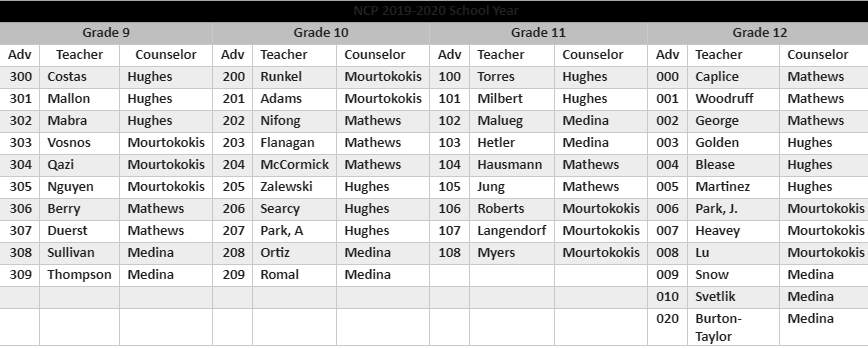table div table+table+table+table+table+table+table+table+table+table+table+table+table+table+table+table+table+table+table+table+table+table+table+table+table+table+table+table+table+table+table+table+table+table+table+table+table+table+table+table+table+table+table+table+table+table+table+table+table+table+table div table{width:100%;padding:0}table div table+table+table+table+table+table+table+table+table+table+table+table+table+table+table+table+table+table+table+table+table+table+table+table+table+table+table+table+table+table+table+table+table+table+table+table+table+table+table+table+table+table+table+table+table+table+table+table+table+table+table div table img{width:96.23%;padding:0;float:none}table div table+table+table+table+table+table+table+table+table+table+table+table+table+table+table+table+table+table+table+table+table+table+table+table+table+table+table+table+table+table+table+table+table+table+table+table+table+table+table+table+table+table+table+table+table+table+table+table+table+table+table div table td{width:100%;padding:0 1.88% 18px}/* styles */# New to Parent Portal?

## Three easy steps to sign up!

1. Obtain your student's PIN, which should be on your acceptance letter or school welcome letter.
2. Create an account HERE.
3. Link a student to your account.
 1 Obtain your student's PIN, which should be on your acceptance letter or school welcome letter.
 2 Create an account HERE.
 3 Link a student to your account.

PINs can only be provided to the parent/guardian in person or by mail to the address on file. They cannot be provided by phone or email, or to a student. To request your PIN, please contact Sonia Fernandez in the Counseling Office.

 table div table+table+table+table+table+table+table+table+table+table+table+table+table+table+table+table+table+table+table+table+table+table+table+table+table+table+table+table+table+table+table+table+table+table+table+table+table+table+table+table+table+table+table+table+table+table+table+table+table+table+table+table+table div table{width:100%;padding:0}table div table+table+table+table+table+table+table+table+table+table+table+table+table+table+table+table+table+table+table+table+table+table+table+table+table+table+table+table+table+table+table+table+table+table+table+table+table+table+table+table+table+table+table+table+table+table+table+table+table+table+table+table+table div table img{width:96.23%;padding:0;float:none}table div table+table+table+table+table+table+table+table+table+table+table+table+table+table+table+table+table+table+table+table+table+table+table+table+table+table+table+table+table+table+table+table+table+table+table+table+table+table+table+table+table+table+table+table+table+table+table+table+table+table+table+table+table div table td{width:100%;padding:0 1.88% 18px}/* styles */# FON Connect

Friends of Northside uses a customer/constituent relationship management (CRM) system known as FON Connect that allows our parent volunteer organization to work from one database for events. It is not a CPS database and is completely different and separate from the CPS Parent Portal and Northside's EPay website.

FON Connect also serves as the NCP Family Directory and allows users to review their donation history, update their contact information, register for upcoming events, and more. Each parent has a separate account. Transactions, including donations, are recorded in the account of the parent who initiated the transaction or donation.

If you’re new to FON Connect, you'll need to set up a login and password. To do so:

▪ Click HERE.
▪ Click on “Forgot Password?”
▪ Enter your email address.
▪ Check your email for the link to create a login name and password.
 ▪ Click HERE.
 ▪ Click on “Forgot Password?”
 ▪ Enter your email address.
 ▪ Check your email for the link to create a login name and password.

Once you have set up your FON Connect account, keep your login and password in a safe, convenient place because they will be your keys to the database throughout your years at Northside.

 table div table+table+table+table+table+table+table+table+table+table+table+table+table+table+table+table+table+table+table+table+table+table+table+table+table+table+table+table+table+table+table+table+table+table+table+table+table+table+table+table+table+table+table+table+table+table+table+table+table+table+table+table+table+table+table div table{width:100%;padding:0}table div table+table+table+table+table+table+table+table+table+table+table+table+table+table+table+table+table+table+table+table+table+table+table+table+table+table+table+table+table+table+table+table+table+table+table+table+table+table+table+table+table+table+table+table+table+table+table+table+table+table+table+table+table+table+table div table img{width:96.23%;padding:0;float:none}table div table+table+table+table+table+table+table+table+table+table+table+table+table+table+table+table+table+table+table+table+table+table+table+table+table+table+table+table+table+table+table+table+table+table+table+table+table+table+table+table+table+table+table+table+table+table+table+table+table+table+table+table+table+table+table div table td{width:100%;padding:0 1.88% 18px}/* styles */# NCP Carpool Connection!

If you're interested in carpooling to school, add your address to the 2019-2020 NCP Carpool map and reach out to contact a potential carpool family on the map. Find the map HERE or on the Welcome page of FON Connect.

# Attention, Parents of CTA Commuters

Did you know that the #82 and #92 buses pick up students in front of Northside at the end of the school day? The Kimball Brown line station is also close to school at Kimball and Lawrence.

These are just a few of the ways students can use public transportation to commute to school. More information on routes for your student can be found at the CTA website HERE.

 table div table+table+table+table+table+table+table+table+table+table+table+table+table+table+table+table+table+table+table+table+table+table+table+table+table+table+table+table+table+table+table+table+table+table+table+table+table+table+table+table+table+table+table+table+table+table+table+table+table+table+table+table+table+table+table+table+table+table div table{width:100%;padding:0}table div table+table+table+table+table+table+table+table+table+table+table+table+table+table+table+table+table+table+table+table+table+table+table+table+table+table+table+table+table+table+table+table+table+table+table+table+table+table+table+table+table+table+table+table+table+table+table+table+table+table+table+table+table+table+table+table+table+table div table img{width:96.23%;padding:0;float:none}table div table+table+table+table+table+table+table+table+table+table+table+table+table+table+table+table+table+table+table+table+table+table+table+table+table+table+table+table+table+table+table+table+table+table+table+table+table+table+table+table+table+table+table+table+table+table+table+table+table+table+table+table+table+table+table+table+table+table div table td{width:100%;padding:0 1.88% 18px}/* styles */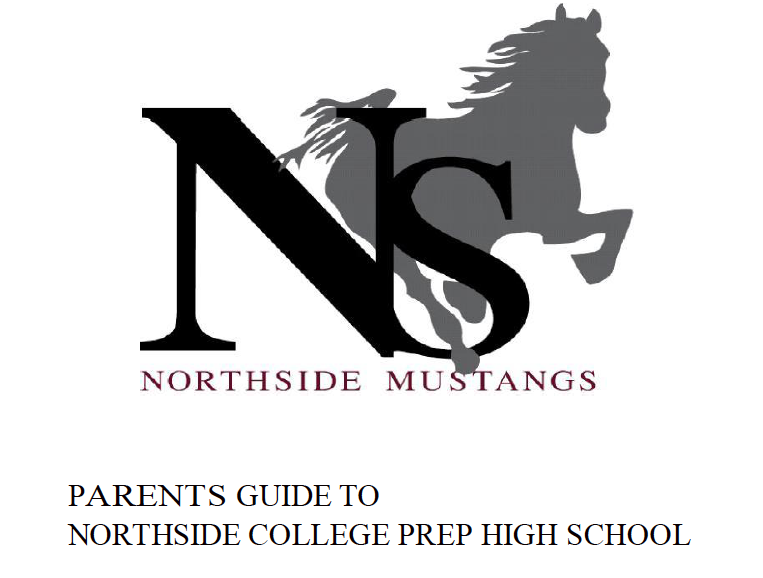# Navigating Northside

Navigating Northside, our parent handbook, is available on the school website under the "Parents" tab. Click HERE to access it directly.

 table div table+table+table+table+table+table+table+table+table+table+table+table+table+table+table+table+table+table+table+table+table+table+table+table+table+table+table+table+table+table+table+table+table+table+table+table+table+table+table+table+table+table+table+table+table+table+table+table+table+table+table+table+table+table+table+table+table+table+table+table div table{width:100%;padding:0}table div table+table+table+table+table+table+table+table+table+table+table+table+table+table+table+table+table+table+table+table+table+table+table+table+table+table+table+table+table+table+table+table+table+table+table+table+table+table+table+table+table+table+table+table+table+table+table+table+table+table+table+table+table+table+table+table+table+table+table+table div table img{width:96.23%;padding:0;float:none}table div table+table+table+table+table+table+table+table+table+table+table+table+table+table+table+table+table+table+table+table+table+table+table+table+table+table+table+table+table+table+table+table+table+table+table+table+table+table+table+table+table+table+table+table+table+table+table+table+table+table+table+table+table+table+table+table+table+table+table+table div table td{width:100%;padding:0 1.88% 18px}/* styles */# Give Back to Northside

Purchase school and office supplies at Office Depot and OfficeMax and Northside can receive 5% back in credits or FREE supplies. Simply provide our school ID 70031171 at checkout, online, or in stores. There’s no cost to you!

 table div table+table+table+table+table+table+table+table+table+table+table+table+table+table+table+table+table+table+table+table+table+table+table+table+table+table+table+table+table+table+table+table+table+table+table+table+table+table+table+table+table+table+table+table+table+table+table+table+table+table+table+table+table+table+table+table+table+table+table+table+table+table+table div table{width:100%;padding:0}table div table+table+table+table+table+table+table+table+table+table+table+table+table+table+table+table+table+table+table+table+table+table+table+table+table+table+table+table+table+table+table+table+table+table+table+table+table+table+table+table+table+table+table+table+table+table+table+table+table+table+table+table+table+table+table+table+table+table+table+table+table+table+table div table img{width:96.23%;padding:0;float:none}table div table+table+table+table+table+table+table+table+table+table+table+table+table+table+table+table+table+table+table+table+table+table+table+table+table+table+table+table+table+table+table+table+table+table+table+table+table+table+table+table+table+table+table+table+table+table+table+table+table+table+table+table+table+table+table+table+table+table+table+table+table+table+table div table td{width:100%;padding:0 1.88% 18px}/* styles */table.module-63{width:66.42%;padding:0}table div table+table+table+table+table+table+table+table+table+table+table+table+table+table+table+table+table+table+table+table+table+table+table+table+table+table+table+table+table+table+table+table+table+table+table+table+table+table+table+table+table+table+table+table+table+table+table+table+table+table+table+table+table+table+table+table+table+table+table+table+table+table+table+table div table{width:66.42%;float:none;margin-left:auto;margin-right:auto;padding:0}table div table+table+table+table+table+table+table+table+table+table+table+table+table+table+table+table+table+table+table+table+table+table+table+table+table+table+table+table+table+table+table+table+table+table+table+table+table+table+table+table+table+table+table+table+table+table+table+table+table+table+table+table+table+table+table+table+table+table+table+table+table+table+table+table div table a{border:0 none;text-decoration:none}table div table+table+table+table+table+table+table+table+table+table+table+table+table+table+table+table+table+table+table+table+table+table+table+table+table+table+table+table+table+table+table+table+table+table+table+table+table+table+table+table+table+table+table+table+table+table+table+table+table+table+table+table+table+table+table+table+table+table+table+table+table+table+table+table div table img{width:100%!important;border:0 none;text-decoration:none}table div table+table+table+table+table+table+table+table+table+table+table+table+table+table+table+table+table+table+table+table+table+table+table+table+table+table+table+table+table+table+table+table+table+table+table+table+table+table+table+table+table+table+table+table+table+table+table+table+table+table+table+table+table+table+table+table+table+table+table+table+table+table+table+table div table td{width:100%;padding:0}/* styles */

# Support Northside - Shop AmazonSmile

AmazonSmile is a simple and automatic way to support Northside every time you shop.

Click HERE to read about AmazonSmile. Click HERE for detailed sign-up instructions or skip the instructions and sign up HERE.

Please note that our AmazonSmile name is Friends of "North" "Side" (North Side - two words at AmazonSmile).

 table div table+table+table+table+table+table+table+table+table+table+table+table+table+table+table+table+table+table+table+table+table+table+table+table+table+table+table+table+table+table+table+table+table+table+table+table+table+table+table+table+table+table+table+table+table+table+table+table+table+table+table+table+table+table+table+table+table+table+table+table+table+table+table+table+table+table div table{width:100%;padding:0}table div table+table+table+table+table+table+table+table+table+table+table+table+table+table+table+table+table+table+table+table+table+table+table+table+table+table+table+table+table+table+table+table+table+table+table+table+table+table+table+table+table+table+table+table+table+table+table+table+table+table+table+table+table+table+table+table+table+table+table+table+table+table+table+table+table+table div table img{width:96.23%;padding:0;float:none}table div table+table+table+table+table+table+table+table+table+table+table+table+table+table+table+table+table+table+table+table+table+table+table+table+table+table+table+table+table+table+table+table+table+table+table+table+table+table+table+table+table+table+table+table+table+table+table+table+table+table+table+table+table+table+table+table+table+table+table+table+table+table+table+table+table+table div table td{width:100%;padding:0 1.88% 18px}/* styles */# Follow Us on Social Media

▪ NCP's Official Facebook Page
▪ Northside Prep Parents Facebook Page, a closed group for parents, by parents, to share their knowledge of Northside
▪ NCP's Twitter Page
▪ Friends of Northside Facebook Page, for news everyone can view
 ▪ Northside Prep Parents Facebook Page, a closed group for parents, by parents, to share their knowledge of Northside
 ▪ Friends of Northside Facebook Page, for news everyone can view
 table div table+table+table+table+table+table+table+table+table+table+table+table+table+table+table+table+table+table+table+table+table+table+table+table+table+table+table+table+table+table+table+table+table+table+table+table+table+table+table+table+table+table+table+table+table+table+table+table+table+table+table+table+table+table+table+table+table+table+table+table+table+table+table+table+table+table+table+table div table{width:100%;padding:0}table div table+table+table+table+table+table+table+table+table+table+table+table+table+table+table+table+table+table+table+table+table+table+table+table+table+table+table+table+table+table+table+table+table+table+table+table+table+table+table+table+table+table+table+table+table+table+table+table+table+table+table+table+table+table+table+table+table+table+table+table+table+table+table+table+table+table+table+table div table img{width:96.23%;padding:0;float:none}table div table+table+table+table+table+table+table+table+table+table+table+table+table+table+table+table+table+table+table+table+table+table+table+table+table+table+table+table+table+table+table+table+table+table+table+table+table+table+table+table+table+table+table+table+table+table+table+table+table+table+table+table+table+table+table+table+table+table+table+table+table+table+table+table+table+table+table+table div table td{width:100%;padding:0 1.88% 18px}/* styles */To view this message in your browser, click HERE.

Seniors, click HERE for the Class of 2020 web page.

Northside’s website can be found HERE.

Friends of Northside's website can be found HERE.

To see all volunteer opportunities on one page, click HERE.

To ensure delivery of This Week at Northside, please add Thisweek@friendsofnorthside.org to your address book.

Please do not reply to this e-mail. Send your questions or comments to Friends of Northside.

# Do you have news or team results to share with the Northside parent community?

## Click HERE to submit information.

 table div table+table+table+table+table+table+table+table+table+table+table+table+table+table+table+table+table+table+table+table+table+table+table+table+table+table+table+table+table+table+table+table+table+table+table+table+table+table+table+table+table+table+table+table+table+table+table+table+table+table+table+table+table+table+table+table+table+table+table+table+table+table+table+table+table+table+table+table+table+table div table{width:100%;padding:0}table div table+table+table+table+table+table+table+table+table+table+table+table+table+table+table+table+table+table+table+table+table+table+table+table+table+table+table+table+table+table+table+table+table+table+table+table+table+table+table+table+table+table+table+table+table+table+table+table+table+table+table+table+table+table+table+table+table+table+table+table+table+table+table+table+table+table+table+table+table+table div table img{width:96.23%;padding:0;float:none}table div table+table+table+table+table+table+table+table+table+table+table+table+table+table+table+table+table+table+table+table+table+table+table+table+table+table+table+table+table+table+table+table+table+table+table+table+table+table+table+table+table+table+table+table+table+table+table+table+table+table+table+table+table+table+table+table+table+table+table+table+table+table+table+table+table+table+table+table+table+table div table td{width:100%;padding:0 1.88% 18px}/* styles *//* styles */